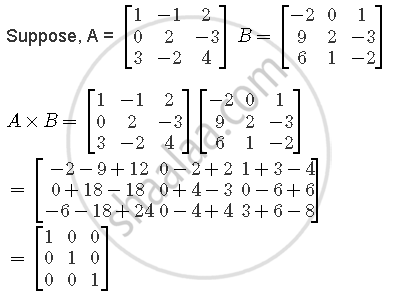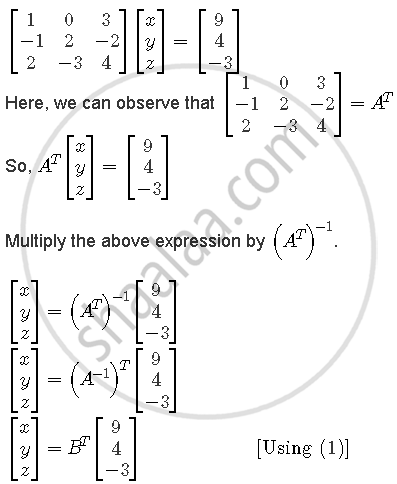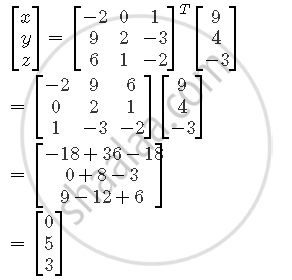Share

# Use Product Matrix[(1,-1,2),(0,2,-3),(3,-2,4)][(-2,0,1),(9,2,-3),(6,1,-2)] To Solve the System of Equations X + 3z = 9, −X + 2y − 2z = 4, 2x − 3y + 4z = −3 - CBSE (Science) Class 12 - Mathematics

#### Question

Use product [(1,-1,2),(0,2,-3),(3,-2,4)][(-2,0,1),(9,2,-3),(6,1,-2)] to solve the system of equations x + 3z = 9, −x + 2y − 2z = 4, 2x − 3y + 4z = −3

#### SolutionSince, A × B = I,

∴ B = A−1 .....(1)

Now, the given system of equations is

x + 3z = 9

−x + 2y − 2z = 4

2x − 3y + 4z = −3

This can also be represented as,Hence, x = 0, y = 5 and z = 3.

Is there an error in this question or solution?

#### Video TutorialsVIEW ALL 

Solution Use Product Matrix[(1,-1,2),(0,2,-3),(3,-2,4)][(-2,0,1),(9,2,-3),(6,1,-2)] To Solve the System of Equations X + 3z = 9, −X + 2y − 2z = 4, 2x − 3y + 4z = −3 Concept: Types of Matrices.
S